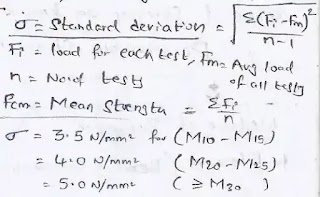# What is the additional property of concrete? ~ Civil Experiences

Property of concrete its seem like the normal topic but useful during planning, designing, construction and also in repair. Like grade of concrete...

Property of concrete seems like a normal topic but useful during planning, designing, construction and also in repair

Like grade of concrete, mix design, unit weight of materials, strength etc so I provide here a short note for the property of concrete

## Property of concrete

In the property of  concrete lots of varies factors come in civil engineering but I try to provide useful point like Top 8 Property of concrete so that you don't feel a burden because you are family of Civil Experiences

### Top 8 Property of concrete as per IS 456:2000

1. What is the minimum grade of concrete?

• A minimum grade of concrete for R.C.C. (reinforced cement concrete) for any construction work is M20 in normal condition and M30 in seawater condition
• You can use a higher grade of concrete as per structure requirement & condition of the environmental condition but not less than given in IS 456:2000 which I also describe above

2. What is the unit weight of RCC?

• Unit weight of R.C.C is 25 KN/m3
• In each estimation of materials need unit weight without that estimation is not possible so you have knowledge about unit weight of each and every material which related to the civil fieldwork on site

3. What is characteristic compressive strength (fck)?

• We conduce varies tests/Specimens for concrete to calculate fck value
• Hence for design purpose, we fix certain fck values by using a formula which given in IS 456:2000 which use in the design grade of the concrete mix as per required
fck = fcm - 1.65𝝈
•characteristic compressive strength (fck)

4. What is Flexural and Split tensile strength (fcr)?

• fcr = 0.7√fck
5. What is the Modulus of elasticity (Ec)?

• its also called "Initial tangent modulus" or short term elastic modulus
• Ec = 5000√fck
6. Shrinkage (Volumetric) strain in concrete = 0.0003

7. Creep of concrete ⊖

• It defined as the increase in deformation with time under constant load
• Creep coefficient ⊖ = (creep strain/elastic coefficient)

• Where ⊖ is 2.2 for 7 days, 1.6 for 28 days and 1.1 for 1 year time
• Long term modulus of elasticity = Short term elasticity/(1 + ⊖) or Ec/(1 + ⊖)

8. Classification of concrete for R.C.C

• Ordinary concrete is varying from M10 to M20 grade of concrete
• Standard concrete is varying from M25 to M55 grade of concrete or mix design of concrete
• High strength concrete is greater than M60
• NOTE: max cement content = 450Kg/m3

### You may like these posts

1. To insert a code use <i rel="pre">code_here</i>
2. To insert a quote use <b rel="quote">your_qoute</b>
3. To insert a picture use <i rel="image">url_image_here</i>